Chapter 11: Functions

# 11.6 Compound Interest

An application of exponential functions is compound interest. When money is invested in an account (or given out on loan), a certain amount is added to the balance. The money added to the balance is called interest. Once that interest is added, the balance will earn more interest during the next compounding period. This idea of earning interest on interest is called compound interest.

For example, if you invest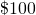at 10% interest compounded annually, after one year, you will earn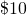in interest, giving you a new balance of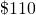. The next year, you will earn another 10% or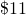, giving you a new balance of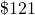. The third year, you will earn another 10% or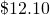, giving you a new balance of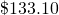. This pattern will continue each year until you close the account.

There are several ways interest can be paid. The first way, as described above, is compounded annually. In this model, the interest is paid once per year. But interest can be compounded more often. Some common compounds include compounded semiannually (twice per year), quarterly (four times per year), monthly (12 times per year), weekly (52 times per year), or even daily (365 times per year).

When interest is compounded in any of these ways, we can calculate the balance after any amount of time using the following formula:

## Compound Interest Formula: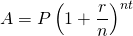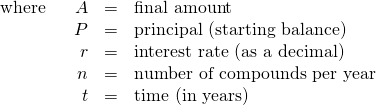The following examples will illustrate the use of the compound interest formula.

Example 11.6.1

If you take a car loan for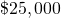with an interest rate of 6.5% compounded quarterly, no payments required for the first five years, what will your balance be at the end of those five years?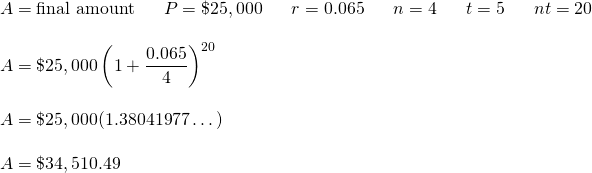Example 11.6.2

What principal will amount to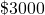if invested at 6.5% compounded weekly for 4 years?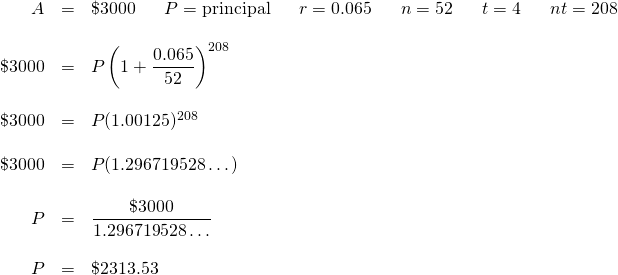# Questions

1. Find each of the following:
1.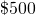invested at 4% compounded annually for 10 years
2.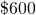invested at 6% compounded annually for 6 years
3.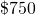invested at 3% compounded annually for 8 years
4.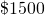invested at 4% compounded semiannually for 7 years
5.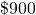invested at 6% compounded semiannually for 5 years
6.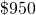invested at 4% compounded semiannually for 12 years
7.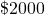invested at 5% compounded quarterly for 6 years
8.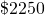invested at 4% compounded quarterly for 9 years
9.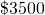invested at 6% compounded quarterly for 12 years
2. If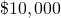is left in a bank savings account drawing 4% interest, compounded quarterly for 10 years, what is the balance at the end of that time?
3. If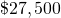is invested in an account earning 6% interest compounded monthly, what is the balance at the end of 9 years?
4. Suppose that you lend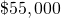at 10% compounded monthly. If the debt is repaid in 18 months, what is the total owed at the time of repayment?
5. What principal will amount to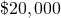if it is invested at 6% interest compounded semiannually for 5 years?
6. What principal will amount to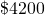if it is invested at 4% interest compounded quarterly for 5 years?